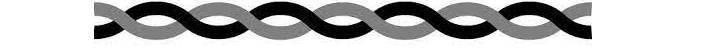# Pair Class in Java

The Java Pair class is used to store two related objects as a single unit. The class is typically used when a method needs to return multiple values, and it is often used in combination with other libraries such as the JavaFX framework. The two objects stored in a Pair instance are called the left and right values. A Pair instance is usually created using the Pair.of method, which creates a new instance of the Pair class with the specified left and right values.

## Java Pair Class example

import javafx.util.Pair; public class Main { public static void main(String[] args) { Pair<String, Integer> pair = Pair.of("Depp", 30);// Create a pair String name = pair.getKey(); Integer age = pair.getValue(); System.out.println("Name: " + name + ", Age: " + age); } } //Output: Name: Depp, Age: 30

In the above example, create a Pair instance using the Pair.of method, which creates a new instance of the Pair class with the specified left and right values. Then use the getKey and getValue methods to access the left and right values of the pair. Note that the javafx.util.Pair class is part of the JavaFX framework, and it is typically used in applications that make use of JavaFX for building user interfaces.

## Immutable Pair Class and Mutable Pair Class

An "Immutable Pair Class" is a Pair class where the left and right values cannot be changed once they are set. This means that once you have created an instance of an immutable Pair class, you cannot modify the values stored in the pair.

A "Mutable Pair Class", on the other hand, is a Pair class where the left and right values can be changed after they are set. This means that you can create an instance of a mutable Pair class and then later modify the values stored in the pair.

For example, the java.util.AbstractMap.SimpleImmutableEntry class is an example of an immutable Pair class, while the java.util.AbstractMap.SimpleEntry class is an example of a mutable Pair class.

Whether you choose to use an immutable or a mutable Pair class will depend on your application requirements and the desired behavior of the Pair instances in your code. If you need to ensure that the values stored in the Pair instance do not change, you would use an immutable Pair class. If you need to be able to change the values stored in the Pair instance, you would use a mutable Pair class.

## Different types of Java Pair classesThere are several different types of Pair classes in Java, some of which are part of the standard Java library and others that are part of different libraries or frameworks. Here are a few of the most common Pair classes:

1. java.util.AbstractMap.SimpleEntry: This is an implementation of the java.util.Map.Entry interface that represents a key-value pair. It is part of the standard Java library and can be used in any Java application.
2. java.util.AbstractMap.SimpleImmutableEntry: This is another implementation of the java.util.Map.Entry interface that represents an immutable key-value pair. It is also part of the standard Java library.
3. javafx.util.Pair: This is a Pair class that is part of the JavaFX framework. It is used in applications that make use of JavaFX for building user interfaces.
4. org.jooq.util.Pair: This is a Pair class that is part of the jOOQ library, which is a Java library for accessing relational databases.
5. org.apache.commons.lang3.tuple.Pair: This is a Pair class that is part of the Apache Commons Lang library, which is a set of Java utility classes for common programming tasks.

These are just a few examples of the different types of Pair classes available in Java. Depending on the needs of your application, you may choose to use one of these classes or create your own custom Pair class.

## javafx.util.Pair class methods (summary)

Here are some of the important methods of the javafx.util.Pair class:

1. getKey(): returns the left value of the pair.
2. getValue(): returns the right value of the pair.
3. setKey(Object key): sets the left value of the pair.
4. setValue(Object value): sets the right value of the pair.
5. hashCode(): returns a hash code value for the pair.
6. equals(Object o): compares this pair to the specified object for equality.
7. toString(): returns a string representation of the pair.